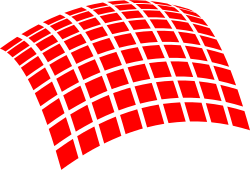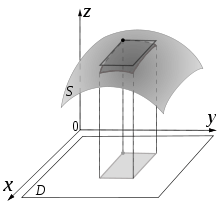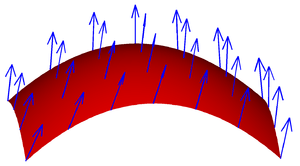# Surface integral

In mathematics, a surface integral is a generalization of multiple integrals to integration over surfaces. It can be thought of as the double integral analog of the line integral. Given a surface, one may integrate over its scalar fields (that is, functions which return scalars as values), and vector fields (that is, functions which return vectors as values).

Surface integrals have applications in physics, particularly with the theories of classical electromagnetism.The definition of surface integral relies on splitting the surface into small surface elements.An illustration of a single surface element. These elements are made infinitesimally small, by the limiting process, so as to approximate the surface.

## Surface integrals of scalar fields

To find an explicit formula for the surface integral, we need to parameterize the surface of interest, S, by considering a system of curvilinear coordinates on S, like the latitude and longitude on a sphere. Let such a parameterization be x(s, t), where (s, t) varies in some region T in the plane. Then, the surface integral is given bywhere the expression between bars on the right-hand side is the magnitude of the cross product of the partial derivatives of x(s, t), and is known as the surface element. The surface integral can also be expressed in the equivalent formwhere g is the determinant of the first fundamental form of the surface mapping x(s, t).

For example, if we want to find the surface area of the graph of some scalar function, say, we havewhere. So that, and. So,which is the standard formula for the area of a surface described this way. One can recognize the vector in the second line above as the normal vector to the surface.

Note that because of the presence of the cross product, the above formulas only work for surfaces embedded in three-dimensional space.

This can be seen as integrating a Riemannian volume form on the parameterized surface, where the metric tensor is given by the first fundamental form of the surface.

## Surface integrals of vector fieldsA vector field on a surface

Consider a vector field v on S, that is, for each x in S, v(x) is a vector.

The surface integral can be defined component-wise according to the definition of the surface integral of a scalar field; the result is a vector. This applies for example in the expression of the electric field at some fixed point due to an electrically charged surface, or the gravity at some fixed point due to a sheet of material.

Alternatively, if we integrate the normal component of the vector field, the result is a scalar. Imagine that we have a fluid flowing through S, such that v(x) determines the velocity of the fluid at x. The flux is defined as the quantity of fluid flowing through S per unit time.

This illustration implies that if the vector field is tangent to S at each point, then the flux is zero, because the fluid just flows in parallel to S, and neither in nor out. This also implies that if v does not just flow along S, that is, if v has both a tangential and a normal component, then only the normal component contributes to the flux. Based on this reasoning, to find the flux, we need to take the dot product of v with the unit surface normal n to S at each point, which will give us a scalar field, and integrate the obtained field as above. We find the formulaThe cross product on the right-hand side of this expression is a (not necessarily unital) surface normal determined by the parametrization.

This formula defines the integral on the left (note the dot and the vector notation for the surface element).

We may also interpret this as a special case of integrating 2-forms, where we identify the vector field with a 1-form, and then integrate its Hodge dual over the surface. This is equivalent to integratingover the immersed surface, whereis the induced volume form on the surface, obtained by interior multiplication of the Riemannian metric of the ambient space with the outward normal of the surface.

## Surface integrals of differential 2-forms

Letbe a differential 2-form defined on the surface S, and letbe an orientation preserving parametrization of S within D. Changing coordinates fromto, the differential forms transform asSotransforms to, wheredenotes the determinant of the Jacobian of the transition function fromto. The transformation of the other forms are similar.

Then, the surface integral of f on S is given bywhereis the surface element normal to S.

Let us note that the surface integral of this 2-form is the same as the surface integral of the vector field which has as components,and.

## Theorems involving surface integrals

Various useful results for surface integrals can be derived using differential geometry and vector calculus, such as the divergence theorem, and its generalization, Stokes' theorem.

Let us notice that we defined the surface integral by using a parametrization of the surface S. We know that a given surface might have several parametrizations. For example, if we move the locations of the North Pole and South Pole on a sphere, the latitude and longitude change for all the points on the sphere. A natural question is then whether the definition of the surface integral depends on the chosen parametrization. For integrals of scalar fields, the answer to this question is simple, the value of the surface integral will be the same no matter what parametrization one uses.

For integrals of vector fields things are more complicated, because the surface normal is involved. It can be proven that given two parametrizations of the same surface, whose surface normals point in the same direction, one obtains the same value for the surface integral with both parametrizations. If, however, the normals for these parametrizations point in opposite directions, the value of the surface integral obtained using one parametrization is the negative of the one obtained via the other parametrization. It follows that given a surface, we do not need to stick to any unique parametrization; but, when integrating vector fields, we do need to decide in advance which direction the normal will point to and then choose any parametrization consistent with that direction.

Another issue is that sometimes surfaces do not have parametrizations which cover the whole surface; this is true for example for the surface of a cylinder (of finite height). The obvious solution is then to split that surface in several pieces, calculate the surface integral on each piece, and then add them all up. This is indeed how things work, but when integrating vector fields one needs to again be careful how to choose the normal-pointing vector for each piece of the surface, so that when the pieces are put back together, the results are consistent. For the cylinder, this means that if we decide that for the side region the normal will point out of the body, then for the top and bottom circular parts the normal must point out of the body too.

Lastly, there are surfaces which do not admit a surface normal at each point with consistent results (for example, the Möbius strip). If such a surface is split into pieces, on each piece a parametrization and corresponding surface normal is chosen, and the pieces are put back together, we will find that the normal vectors coming from different pieces cannot be reconciled. This means that at some junction between two pieces we will have normal vectors pointing in opposite directions. Such a surface is called non-orientable, and on this kind of surface one cannot talk about integrating vector fields.